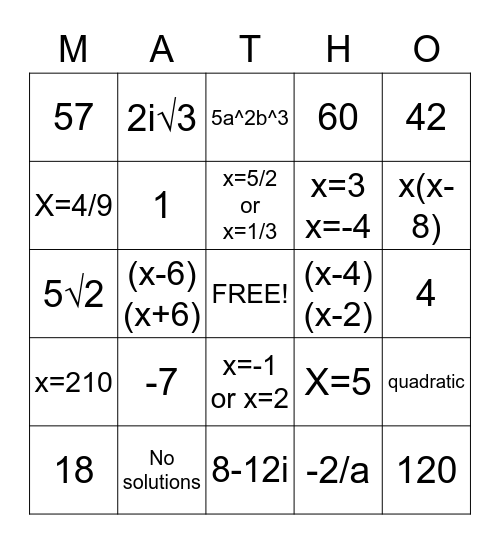# Math SOL ReviewThis bingo card has a free space and 24 words: -7, No solutions, x=5/2 or x=1/3, 18, 42, 57, X=4/9, 2i√3, (x-4)(x-2), x=-1 or x=2, x=3 x=-4, 5√2, X=5, quadratic, 1, -2/a, 4, 5a^2b^3, x(x-8), (x-6)(x+6), 120, x=210, 60 and 8-12i.

## Play Online